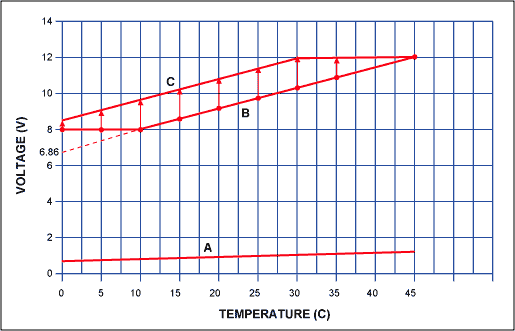# Fan-Control Circuits and Speed Control Guide

### 要約

A linear circuit measures temperature and controls the speed of a cooling fan by generating a variable supply voltage for the fan.

Fan noise is becoming a larger issue as more electronic equipment enters the office and the home. A variable speed fan permits slower, and quieter, fan speed when temperature conditions allow.

Fan-control circuits range from simple switches that boost the fan speed at a certain temperature, to digitally controlled fans with continuously-variable speed. High-speed/low-speed switches are inexpensive, but the sound of sudden speed changes can be annoying. Digitally controlled fans perform well, but those circuits are more costly, and the system must include a serial bus. This application note presents a low cost, self-contained analog circuit for fan-speed control (Figure 1), which is easily adjusted for any desired linear relationship between the fan voltage and temperature (Figure 2, Curves B and C). Actual data points are plotted against the desired voltage in Figure 2.Figure 1. This circuit delivers a continuous and linear fan-control voltage that is proportional to temperature.Figure 2. As described in the text, these curves illustrate voltage outputs vs. temperature for the circuit in Figure 1.

Curve "A" on Figure 2 represents the output of a MAX6605 analog temperature sensor vs. temperature in °C:

Vsensor = 0.0119V/°C × Temp + 0.744V.

Curve "B" relates the fan voltage to temperature and combines a minimum "floor" voltage of 8.0V with a sloping line:

Vfan = 0.114V/°C × Temp + 6.86V.

The floor voltage ensures fan rotation at low temperatures, and above 10°C, the voltage increases with a slope of 0.114V/°C until it reaches full value at 45°C. Simply amplifying the MAX6605 output does not provide the 8V floor voltage, and the gain (9.58 = 0.114/0.0119) needed to obtain the fan-voltage slope is not the same gain (9.22 = 6.86V/0.744V) needed to obtain the y-intercept point.

To transform line "A" into line "B", you must subtract a voltage offset from the temp sensor output and then multiply the result by a constant. That can be accomplished with the circuit of Figure 1, in which you connect the dotted line labeled "reduce the offset." One op amp creates the sloped line, and the second op amp creates the floor voltage. The op amp outputs are connected to transistors in such a way that the op amp demanding a higher output voltage dominates. The following equations let you determine the resistor values:

For the condition R2<<R1,

R2 = R1(AvVtemp0 - Vy-intB)/[(Av-1)(Vref - Vtemp0 + Vy-intB/Av)], and

R3 = R2(Av-1), where

R1 is any reasonable value,

Av = 0.114/0.0119 = 9.58 is the ratio of the desired slope in V/°C to that of the temperature sensor,

Vtemp0 = 0.744V is the temp-sensor voltage at 0°C,

Vy-intB = 6.86V is the y-intercept indicated by the desired (extrapolated) temperature curve, and

Vref = 3.0V is the reference voltage.

Thus, choosing R1 = 301kΩ lets you calculate R2 = 3.158kΩ and R3 = 27.09kΩ. The closest 1% values are 3.16kΩ and 27.0kΩ, respectively.

The following equation lets you calculate the floor voltage:

R5 = R6(Vfloor - Vref)/(Vref), where R6 equals any reasonable value, and

Vfloor = 8V is the desired minimum output voltage.

Thus, choosing R6 = 100kΩ lets you calculate R5 = 169kΩ.

In some cases, the required offset gain is greater than the required slope gain, so you must increase the temperature sensor&'s natural offset. For a desired temperature curve "C" expressed as:

Vfan = (0.114V/°C)(Temp + 8.5V),

the gain (slope) of Av = 9.58 is the same as for line "B", but the required offset gain (8.5V/0.744V = 11.42) is greater. You therefore use the "increase the offset" version of the schematic. The following equation holds for such cases:

R4 = R1(Vy-intC/Av - Vtemp0)/(Vref - Vy-intC/Av) = 20.41kΩ,

where Vy-intC = 8.5V is the intersection of the desired temperature curve with the y axis. For R1 = 301kΩ, the closest available 1% value for R4 is 20.5kΩ.

A similar version of this article appeared in the March 21, 2002 issue of EDN magazine.